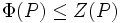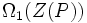# Commutator subgroup lemma for Frattini-in-center cyclic-center p-group

## Statement

Suppose$P$ is a non-Abelian$p$-group with the following two properties:

•$P$ is a Frattini-in-center group: In other words,$P/Z(P)$ is elementary Abelian, or equivalently,$\Phi(P) \le Z(P)$, where$\Phi(P)$ and$Z(P)$ are respectively the Frattini subgroup and the center of$P$.
• The center of$P$ is a cyclic group.

Then, the following are true:

1.$P' = \Omega_1(Z(P))$ is a cyclic subgroup of order$p$. It is the unique minimal normal subgroup as well as the unique minimal characteristic subgroup of$P$.
2. Every element of$P'$ is invariant under all the$p$-automorphisms of$P$. In particular, if$P$ is embedded as a normal subgroup of some$p$-group$Q$, then$P' \le Z(Q)$.
3. If$P$ is a normal subgroup of a$p$-group$Q$ such that$[Q,P] \le Z(P)$, and$E$ is a normal subgroup of$P$ such that$E/P'$ is elementary Abelian, then$[Q,E] \le P'$.

## Proof

### Proof of part (1)

By fact (1),$P'$ is elementary Abelian. Also,$P' \le Z(P)$ since$P/Z(P)$ is elementary Abelian. Thus,$P'$ is a nontrivial elementary Abelian subgroup of the cyclic subgroup$Z(P)$, forcing$P'$ to equal$\Omega_1(Z(P))$, which is cyclic of order$p$.

By fact (2),$\Omega_1(Z(P))$ is normality-large in$P$. Since it is cyclic of prime order, this forces it to be contained in every nontrivial normal subgroup. Thus, it is the unique minimal normal subgroup, and hence also the unique minimal characteristic subgroup.

### Proof of part (2)

Since$P'$ has order$p$, we cannot have any$p$-automorphisms moving around its non-identity elements. Thus, every element of$P'$ is invariant under all the$p$-automorphisms of$P$. In particular, if$P$ is a normal subgroup of a$p$-group$Q$, every element of$P'$ is invariant under all the inner automorphisms of$Q$, so$P' \le Z(Q)$.

(Note: This can also be seen from the fact that$P'$ is normal in$Q$, using that characteristic of normal implies normal, and thus is central, using the fact that minimal normal implies central in nilpotent).

### Proof of part (3)

Since$E \le P$,$[Q,E] \le [Q,P] \le Z(P)$. In particular, every element of$[Q,E]$ commutes with every element of$P$, and thus, with every element of$E$. This yields that for$x \in Q$ and$y,z \in E$, we have:$[x,y][x,z] = [x,yz]$.

In particular, we have, for$x \in Q$ and$y \in E$:$[x,y^p] = [x,y]^p$.

Since by assumption$E/P'$ is elementary Abelian,$y^p \in P'$. We showed in part (2) that$P' \le Z(Q)$, so$y^p \in Z(Q)$, so$[x,y^p] = e$. Thus,$[x,y]^p = e$, so$[Q,E]$ is generated by elements of order$p$. Since$[Q,E] \le Z(P)$ by assumption, we obtain that$[Q,E] \le \Omega_1(Z(P)) = P'$.